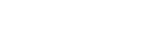# MATH222 - Calculus II

## General

MATH

222

00161709

Lecture

### Description

Integration techniques and applications, inverse functions, topics in analytic geometry, sequences and series, improper integrals, plane curves. Prereq.: MATH 221.

4

4

No

Yes

04

No

## Learning Outcomes

### Outcome

Use integration to find volumes of solids described via cross-section and solids of revolution, to find the lengths of curves, to find the surface area of a surface of revolution and to solve physical problems involving concepts such as work, hydrostatic force, and center of mass.

### Outcome

Calculate integrals exactly by integration by parts, inspection of powers of trigonometric functions, trigonometric substitution, and partial fractions.

### Outcome

Approximate integrals by using the Trapezoidal Rule, Simpson¿s Rule, and Taylor series.

### Outcome

Use analytic methods to evaluate integrals whose integrands have asymptotes or whose interval of integration is infinite.

### Outcome

Sketch curves given by parametric equations or polar equations, and represent given curves as parametric equations or polar equations.

### Outcome

Convert points and equations from polar coordinates to Cartesian coordinates, and vice versa.

### Outcome

Calculate areas, tangents, and lengths related to curves given by parametric or polar equations.

### Outcome

Calculate limits of sequences by algebraic and numerical methods and the Squeeze Theorem.

### Outcome

Identify geometric series, telescoping series, and the harmonic series and test series for convergence by using the integral test, the comparison tests, methods for working with alternating series, the ratio test, and the root test.

### Outcome

Represent a given function as a power series over a suitable interval and find the interval of convergence of a given power series.

### Outcome

Give the formulas and intervals of convergence for selected Taylor series such as the ones for the sine, cosine, inverse tangent, and natural exponential functions.

### Outcome

State and apply named theorems of calculus (Bounded Monotonic Sequence Theorem, Taylor¿s Theorem).

### Outcome

Communicate their knowledge of the principles of Calculus II, both orally (e.g. class discussions) and in writing (e.g. written assessments).

-

## Dependencies

No dependencies720 4th Avenue South
St. Cloud, MN 56301-4498
(320) 308-0121St. Cloud State University
A member of Minnesota State and committed to legal affirmative action, equal opportunity, access and diversity of its campus community (Full Statement).
© 2021 St. Cloud State University